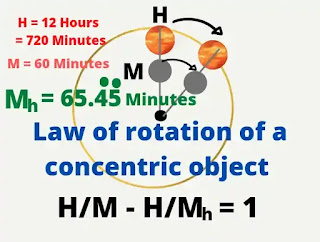# Very important formula for basic Islamic Astronomy 》LAW OF ROTATION OF A CONCENTRIC OBJECT

# Law of rotation of a concentric object :-

The orbital period of two concentric objects and their connecting period always form a definite mathematical equation. If two objects move in the same direction at the same time, They will always be in the same position relative to the center.

If two objects orbiting at  the same direction at different times, then in the period of the orbiting object in the longest time, the amount of orbital number of the orbiting object in less time will be 1 more than the value of the number of their connecting times.

Subject of the new law of Astronomy are

The longest orbiting object is H.

Orbiting in less time M.

And their connection time to the center is Mh.

So the mathematical equation formed by them will be

H/M - H/Mh = 1  ---- ( a )

This is the first new law of Astronomy

Now we get from this equation

Mh = H.M/( H - M ) --- (1)

H = Mh.M/(Mh - M) --- (2)

M = H.Mh/(H+Mh) --- (3)

We get these equations from new law of Astronomy    (a)

Keeping  H =12 hours

= 12×60 = 720 minutes

M = 60 minutes.

We get from  (1)

Mh = H.M/(H-M).

Mh = 720 × 60/( 720 - 60 ) minutes

Mh = 65.454545-- minutes

So we can easily say that the minute hand revolves around the hour cut in 65.4545--------- minutes.

#### Reciprocal motion :Law of rotation of a concentric objects

If the objects H and M were moving in opposite directions to each other, the equation would occur

H/Mh - H/M = 1

This is the second new law of Astronomy.

Now we get from this equation

Mh = H.M/(H+M) --- (4)

H = M.Mh/(M-Mh) --- (5)

M = H.Mh/(H-Mh) --- (6)

We get these equations from new law of Astronomy   (b)

If two objects revolved opposite each other, their connection time would be    Mh  relative to their center. We get from  (4)

Mh = H.M/(H+M)

= 720 × 60/(720 + 60)

= 55.384615 ------ minutes

That is, if two objects revolve in opposite directions to each other, then their connection period will be 55.384615-----  minutes.

With this formula we can determine the actual rotation period of the earth.

## Determining the correct rotation period of the earth : -

The general principle of surveying to work from the whole to the part. So I chose a larger value first.

We know that the first point of Aries ( and therefore first point of Libra also ) moves slowly backward ( i.e. ,in the direction opposite to that of the sun's  annual motion among the stars ) along the ecliptic and it would take 813941638698 seconds  ~ 25,793 years to complete one revolution.

The Earth is making a full rotation relative to the first point of Aries in 86164.0905 seconds ( 23 hor 56 min 4.1 sec ).

We get from  (6)

M = H.Mh/(H-Mh)  》》

E = P.Ep/(P - Ep)

= 86164.09962 seconds

~ 86164.0996 seconds

So our earth completes one orbit on its own axis in about 23 hours 56 minutes 4.100 seconds ( Upcoming values ​​up to three decimals ).

### Determine the synodic month :-

We know that the moon takes 27.32166 days
( 2360591.5 seconds ) to complete one orbit around the earth. And so on, we know that The sun takes 365.256363 days
( 31558149.5 seconds ) to complete one orbit around the earth.
Taking  S = 365.256363d
= 365d 6hr 9min 9.5sec
=31558149.5 seconds
M = 27.321661 days
= 27d 7hr 43min 11.5sec
= 2360591.5 seconds

The moon orbits our earth relative to the sun in  Ms seconds.
We get from  ( 1 )
Mh = H.M/( H - M )  》》
Ms = S.M/(S-M)
= 2551442.8 seconds
= 29d 12hr 44min 03sec
= 29.53059 days

So, the mean length of the Synodic month is 29 days 12 hours 44 minutes 03 seconds

#### Determining the period of rotation of the Nodes :-

We know that these nodes are not fixed points but have a retrograde motion along the ecliptic.
Let N  be the revolution period of the Nodes.
Revolution period of the Moon M = 27.32166 days
The Moon orbits our earth relative to the Nodes Mn = 27.2122 days
We get from equation no (5)
H = M.Mh/(M-Mh) 》》
N = N.Mn/(M-Mn)
from this we get
N = 6792.2755 days
= 18.5966 years

#### These Nodes are completing a revolution period in about 18.60 years ( Upcoming values ​​up to two decimals )

In this way the complex issues of astronomy can be solved very easily by these equations.